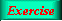Lecture 4 - Page 12 : 34
 Functional Programming in SchemeHigher-order Functions * Introduction to higher-order functions Higher-order functions Some simple and general higher-order functions Linear search in lists Generation of list selectors * Mapping and filtering Classical higher-order functions: Overview Mapping The mapping function Examples of mapping Filtering The filtering function Examples of filtering * Reduction and zipping Reduction The reduction functions Accumulation Zipping The zipping function * Currying The idea of currying Currying in Scheme Examples of currying Ad hoc currying in Scheme (1) Ad hoc currying in Scheme (2) * Web related higher-order functions HTML mirror generation HTML mirror usage examples Making tables with the real mirror Tables with higher-order functions HTML element modifications The function simple-html-table The XHTML mirror in LAML Generation of a leq predicate from enumeration
 The filtering function The next item on the agenda is an implementation of filter .
 For practical purposes it is important to have a memory efficient filter function
 ```(define (filter pred lst) (reverse (filter-help pred lst '()))) (define (filter-help pred lst res) (cond ((null? lst) res) ((pred (car lst)) (filter-help pred (cdr lst) (cons (car lst) res))) (else (filter-help pred (cdr lst) res))))```

An implementation of filter which is memory efficient. If the predicate holds on an element of the list (the red fragment) we include the element in the result (the brown fragment). If not (the green fragment), we drop the element from the result (the purple fragment).A straightforward filter function